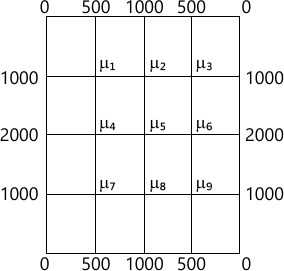MORE IN Engineering Mathematics 3
VTU Computer Science (Semester 3)
Engineering Mathematics 3
January 2013
Total marks: --
Total time: --
INSTRUCTIONS
(1) Assume appropriate data and state your reasons
(2) Marks are given to the right of every question
(3) Draw neat diagrams wherever necessary

1 (a) Find the Fourier series of f(x)=x-x2, -π ≤ x ≤ π. Hence deduce that [ dfrac {1}{1^2}-dfrac {1}{2^2}+dfrac {1}{3^2}- cdots cdots = dfrac {pi^2}{12} ] Is the above deduced series convergent? (Answer in Yes or No)
7 M
1 (b) Define :
i) Half range Fourier sine series of f(x)
ii) Complex form of Fourier series of f(x)
Find the half range cosine series of f(x)=x in 0
7 M
1 (c) Obtain a0, a1, b1 in the Fourier expansion of y, using harmonic analysis for the data given.
 x 0 1 2 3 4 5 y 9 18 24 28 26 20
6 M

2 (a) Find the Fourier transform of [ egin {align*} f(x)&=1-x^2 & for & |x|le 1 \ &=0 &for & |x|>1 end{align*} ] Hence evaluate [ int^{infty}_{0}dfrac {x cos x -sin x}{x^3}cos left ( dfrac {x}{2} ight )dx ]
7 M
2 (b) Find the Fourier sine transform of [ dfrac {e^{-ax}}{x} ]
7 M
2 (c) Find the Fourier cosine transform of [ egin{align*} f(x)&=4x, & for &04 end{align*} ]
6 M

3 (a) i) Write down the two dimensional heat flow equation (p d e) in steady state (or two dimesional) Laplace's equation. Just mention.
ii) Solve one dimensional heat equation by the method of separation of variables.
7 M
3 (b) Using D' Alembert's method, solve one dimensional wave equation.
7 M
3 (c) A string is stretched and fastened to two points/apart. Mention is started by displacing the string in the form of y=a sin (πx/l) from which it is released at time t=0. Show that the displacement of any point at a distance x from one end at time t is, [ y(x,t)=a sin left (dfrac {pi x}{l} ight )cos left ( dfrac {pi ct}{l} ight ) ] Start the answer assuming the solution to be
y=(C1 cos(px)+C2sin(px))(C3cos(cpt)+C4sin(cpt))
6 M

4 (a) Fit a linear law, P=mW+C, using the data
 P 12 15 21 25 W 50 70 100 120
6 M
4 (b) Find the best values of a and b by fitting the law V=atb using method of least square for the data,
 V (ft/min) 350 400 500 600 t (min) 61 26 7 26

Use base 10 for algorithm for computations.
7 M
4 (c) Using simplex method,
Maximize Z=5x1+3x2
Subject to, x1 +x2≤2; 5x1+2x2≤10; 3x1+8x2≤12; x1, x≥0.
7 M

5 (a) Use Newton-Raphson method, to find the real root of the equation 3x=(cos x)+1 Take x0=0.6. Perform two iterations.
6 M
5 (b) Apply Gauss-Seidel iteration method to solve equations.
20x+y-2z=17
3x+20y-z=-18
2x-3z+20z=25
Assume initial approximation to be x=y=z=0. Perform three interations.
7 M
5 (c) Using Rayleigh's power method to find the largest eigen values and the corresponding eigen vector of the matrix.
[ A=egin{bmatrix}6 &-2 &2 \-2 &3 &-1 \2 &-1 &3 end{bmatrix} ] Take [1 0 0]T as the initial approximation. Perform four iterations.
7 M

6 (a) Use appropriate interpolating formula to compute y(82) and y(98) for the data
 x 80 85 90 95 100 y 5026 5674 6362 7088 7854
7 M
6 (b) i) For the point (x0, y0) (x1, y1) (x2, y2) mension Lagrage's interpolation formula.
ii) If f(1)=4, f(3)=32, f(4)=55, f(6)=119: find interpolating polynomial by newton's divided difference formula.
7 M
6 (c) Evaluate [ int^{6}_0 dfrac {1}{1+x^2}dx, ] using i) Simpson's 1/3rd rule ii) Simpson's 3/8th rule iii) Weddle's rule using
 x 0 1 2 3 4 5 6 f(x)=1/1+x2 1 0.5 0.2 04 0.0588 0.0385 0.027
6 M

7 (a) Solve the wave equation [ dfrac {partial^2 u}{partial t^2}=4 dfrac {partial^2 u}{partial x^2} ] subject to u(0, t), u(4, t)=0. ut(x,0)=0 and u(x, 0)=x(4-x) by taking h=1, k=0.5 upto four steps.
7 M
7 (b) Solve two dimensional Laplace equation at the pivotal or nodal points of the mesh shown in Fig. Q7(b). To find the initial values assume u4=0. Perform three iterations including computation of initial values.7 M
7 (c) Solve the equation [ dfrac {partial^2 u}{partial t^2}=4 dfrac {partial^2 u}{partial x^2} ] subjects to the conditions u(x, 0)=sinπx, 0 ≤ x ≤ 1; u(0,t)=u(1,t)=0. Carry out computations for two levels, taking h=1/3, k=1/36.
6 M

8 (a) Find the z-transform of, [ dfrac {n}{3^n}+2^nn^2+4cos (n heta)+4^n+8 ]
7 M
8 (b) State and prove i) initial value theorem ii) Final value theorem of z-transforms.
7 M
8 (c) Using the z-transform solve
un+2+4un+1+3unn=3n with u0=0, u1=1.
6 M

More question papers from Engineering Mathematics 3